Q

# Give answer! - Oscillations and Waves - NEET

The oscillation of a body on a smooth horizontal surface is represented by the equation,

X = A cos ( t)

where, X = displacement at time t

= frequency of oscillation

Which one of the following graphs shows correctly the variation of 'a' with 't'?

• Option 1)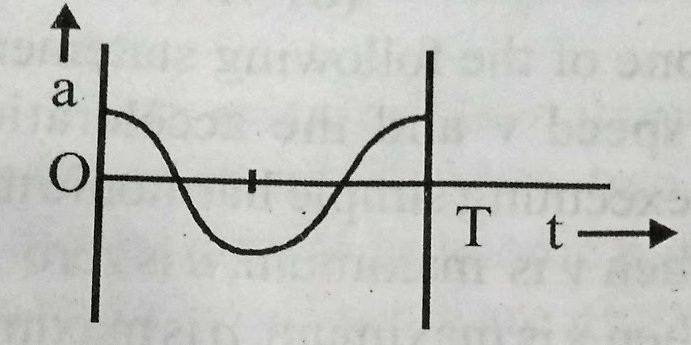• Option 2)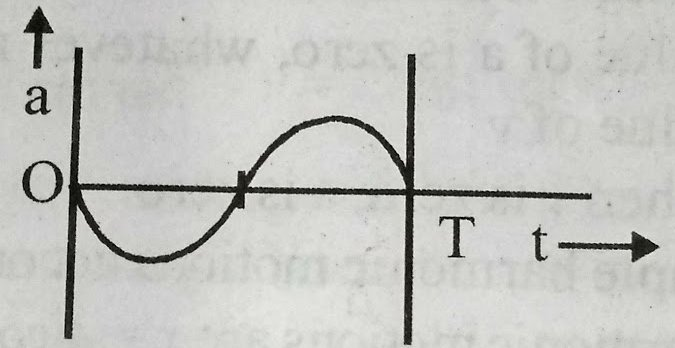• Option 3)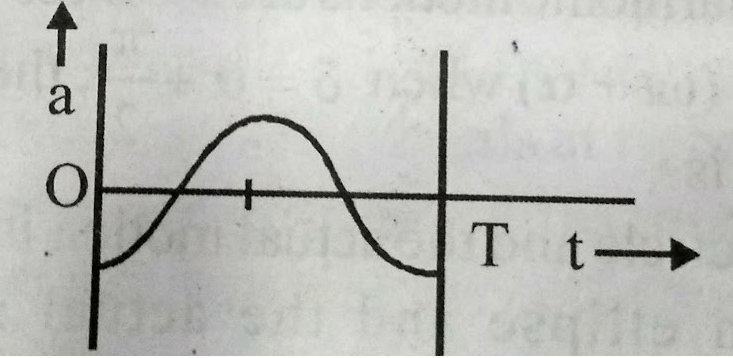• Option 4)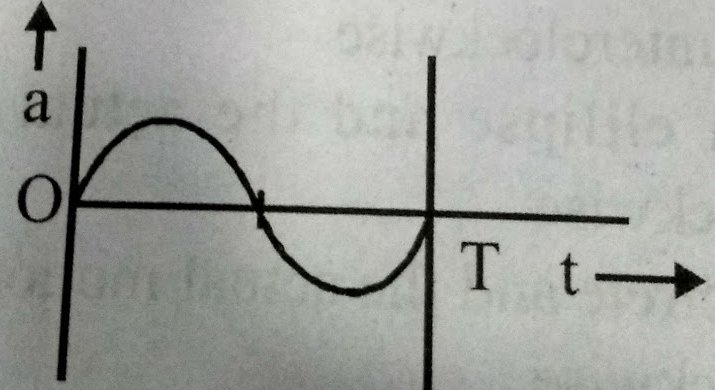113 Views

As discussed

Composition of two SHM in perpendicular direction -

- wherein

Resultant equation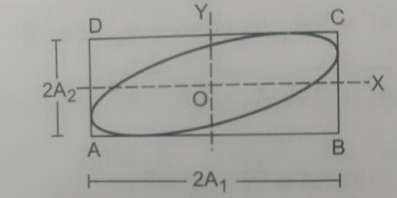a=A coswt

v=-AwSinwt

a=

i.e the variation of a with and correctly shown by graph (3)

Option 1)This option is incorrect

Option 2)This option is incorrect

Option 3)This option is correct

Option 4)This option is incorrect

Exams
Articles
Questions